# Double Replacement Reaction | Examples

Jayashree Raman, Nissa Garcia, Matthew Bergstresser
• Author
Jayashree Raman

Jayashree has taught high school chemistry for over thirty years. She has a bachelor's degree in chemistry and master's degree in English from Madurai University. She has a teaching certification, Bachelor of Education, from University of Delhi.

• Instructor
Nissa Garcia

Nissa has a masters degree in chemistry and has taught high school science and college level chemistry.

• Expert Contributor
Matthew Bergstresser

Matthew has a Master of Arts degree in Physics Education. He has taught high school chemistry and physics for 14 years.

Learn about double replacement reaction definition, or as also known as double displacement reaction. Read about different types, how to do double replacement reactions, and examples. Updated: 09/13/2021

Show

## Double Replacement Reaction Reaction

A double replacement reaction is a type of chemical reaction. The name describes the process very well. Two replacements take place in the reaction. The reaction takes place between two ionic compounds, usually in the form of a solution.

Ionic compounds are neutral, though they are made up of cations (positive ions) and anions (negative ions). A double replacement reaction can be defined as a reaction in which one set of ions of the same charge are exchanged and two new products are formed.

It can be illustrated as follows: a double replacement reaction takes place between two compounds, AB and XY. The double replacement reactions can be shown as

{eq}A^{+}B^{-} + X^{^{+}}Y^{^{-}}\rightarrow A^{+}Y^{-} +X^{+}B^{-} {/eq}

The anion {eq}B^{-} {/eq} goes to {eq}X^{+} {/eq} and the anion {eq}Y^{^{-}} {/eq} goes to {eq}A^{+} {/eq}

Another example showing the charges is: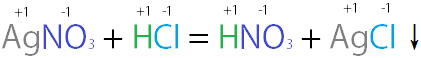### Other Names for Double Replacement Reactions

Other names for double replacement reactions are:

• salt metathesis
• double displacement reactions
• double decomposition reactions (the term used when one of the products is a solid)

### Types of Double Replacement Reactions

Double replacement reactions are of three categories. They are:

• Reactions that produce precipitates
• Reactions that produce molecular compounds
• Reactions that produce effervescence (a gas is evolved as one of the products)

Precipitation Reaction: Double replacement reactions produce precipitates or insoluble solids as one of the products. These reactions are easy to see and classify. For example, lead(II) chloride dissolves moderately in hot water. Potassium sulfate dissolves readily in water at room temperature. To a solution of lead(II) chloride taken in a test tube, a small quantity of potassium sulfate solution is added and there is an immediate precipitate formation. The reaction can be represented as

{eq}PbCl_{2(aq)}+ K_{2}SO_{4(aq)}\rightarrow 2KCl_{(aq)} + PbSO_{4(s)} {/eq}

It can also be represented as {eq}Pb^{2+}(Cl^{-})_{2} + 2K^{+}(SO_{4}))^{^{2-}} \rightarrow \ 2K^{+}Cl^{-}+ Pb^{2+}(SO_{4})^{2-} {/eq}

Another example of a precipitation reaction is the addition of sodium hydroxide solution to ferrous sulfate solution. Iron(II) hydroxide is produced as a precipitate, with the other product being a sodium chloride solution.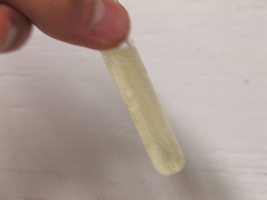{eq}2NaOH_{(aq)}+ FeCl_{2(aq)} \rightarrow 2NaCl_{(aq)} +Fe(OH)_{2(s)} {/eq}

Formation of a molecular compound:

Another form of double replacement reaction is one that gives rise to a molecular compound. Most often, the molecular compound formed is water. So, neutralization reactions are good examples of this type. Neutralization reactions take place between an acid and a base. The essential reaction is between the hydrogen ion of the acid and the hydroxide ion of the base.

{eq}NaOH_{(aq)} + HCl_{(aq) }\rightarrow NaCl_{(aq)} + H_{2}O_{(l))} {/eq}

The hydroxide ion and the hydrogen ion combine to form water. The other ions, sodium and chloride, remain unchanged. They are spectator ions.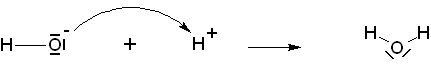Gas formation: An example of a double replacement reaction that results in a gas is the reaction between a carbonate or a hydrogen carbonate and dilute acids. In both cases, carbon dioxide is released as a gaseous product.

{eq}K_{2}CO_{3(s)} + H_{2}SO_{4(aq)}\rightarrow K_{2}SO_{4(aq)} +H_{2}O_{(l)} +CO_{2(g)} {/eq}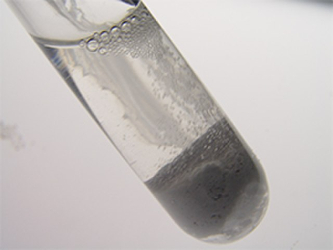{eq}NaHCO_{3(s)} + HCl_{(aq)}\rightarrow NaCl_{(aq)} +H_{2}O_{(l)} +CO_{2(g)} {/eq}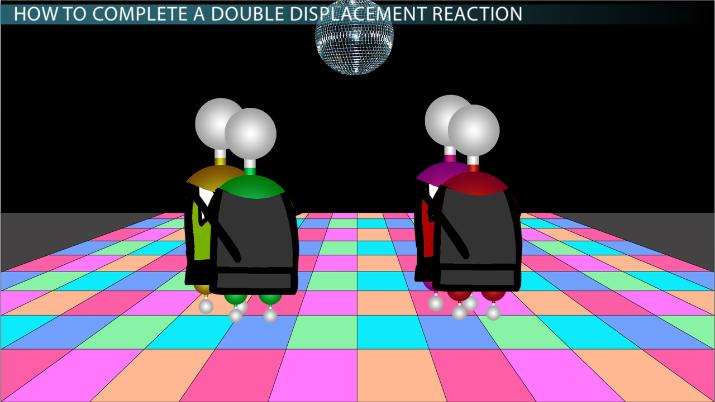An error occurred trying to load this video.

Try refreshing the page, or contact customer support.

Coming up next: Alkanes: Definition, Properties, Formula & Examples

### You're on a roll. Keep up the good work!

Replay
Your next lesson will play in 10 seconds
• 0:01 What Is a Double…
• 0:46 How to Complete a…
• 3:05 Examples of Double…
• 4:06 Types of Double…
• 6:49 Lesson Summary
Save Save

Want to watch this again later?

Timeline
Autoplay
Autoplay
Speed Speed

## How to Do Double Displacement Reactions.

The following points come into play when doing a double replacement reaction:

To unlock this lesson you must be a Study.com Member.

• Activities
• FAQs

## Double Displacement Reactions

Double displacement reactions occur when two aqueous solutions react to form either water, a solid (insoluble compound) or a gas and an aqueous solution. Let's work on predicting and balancing the reactions.

### Problems for More Practice

Write the balanced chemical reaction for each scenario, which includes predicting the products of the reaction.

1) Sodium oxide reacts with silver acetate.

2) Silver nitrate reacts with magnesium chloride.

3) Potassium carbonate reacts with ammonium iodide.

4) Cesium sulfide reacts with sodium hydroxide.

5) Sodium hydroxide reacts with hydrochloric acid.

### Solutions

1) Na2 O + 2AgC2 H3 O2 ⟶ 2NaC2 H3 O2 + Ag2 O

2) 2AgNO3 + MgCl2 ⟶ 2AgCl + Mg(NO3 )2

3) K2 CO3 + 2NH4 I ⟶ 2KI + (NH4)2 CO3

4) Cs2 S + 2NaOH ⟶ 2CsOH + Na2 S

5) NaOH + HCl ⟶ NaCl + H2 O

Notice that in each of these reactions, they're balanced for mass. Reactions 1-4 produce a precipitate, while reaction 5 produces water.

#### What is double displacement reaction with example?

A double displacement reaction takes place between two ionic compounds. Ions are exchanged between them. Only ions of the same charge are exchanged.

An example is HCl + NaOH -> NaCl + H2O

#### What is double displacement formula?

There is a general formula for representing double displacement reactions.

It is

AB + XY -> AY + XB.

A and X have the same number of positive charges and B and Y have the same number of negative charges.

#### What are the 3 types of double displacement reactions?

The three types of double replacement reactions are

• precipitation reaction
• neutralization reaction, in which molecular compound is produced
• gas formation reaction

### Register to view this lesson

Are you a student or a teacher?